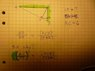# Affine Geometric Problem

• Horse

## Homework Statement

Show that the ratio of areas is proportional to the sides squared:

$$\frac{[ACD]}_{[CDB]}$$ is proportional to $$\frac{AC^2}_{CB^2}$$

Please, see the picture: http://dl.getdropbox.com/u/175564/geo_henry.JPG [Broken].

## Homework Equations

AC = 2 * CB

$$\frac{AD}_{DB}$$ is propotional to $$\frac{[ACD]}_{[CDB]}$$

where [ACD] and [CDB] are areas.

## The Attempt at a Solution

I was unable to prove the relation with pythagoras, so I feel an easier solution. Perhaps, you can prove it somehow with affine geometry.

Last edited by a moderator:

Picture

#### Attachments

•geo.JPG
26.2 KB · Views: 348
Last edited by a moderator:
The basic idea is that if two triangles are similar (same corresponding angles), their corresponding sides will be proportional.

$\angle$ACD = $\angle$CDM - alt. interior angles cut by transversal
$\angle$MDB = $\angle$DAC - complements of congruent angles are congruent
$\angle$ABC = $\angle$ACD - complements of congruent angles are congruent

The statements above show that triangle ACD is similar to triangle CDB.
[ACD] = 1/2 * AD * CD
[CDB] = 1/2 * DB * CD

The two equations above show the proportionality you want.

The statements above show that triangle ACD is similar to triangle CDB.
[ACD] = 1/2 * AD * CD
[CDB] = 1/2 * DB * CD

The two equations above show the proportionality you want.

I am sorry of the blurry image. I wanted to know why the relation is true:

$$\frac{AC^2}_{CB^2}$$ is proportional to $$\frac{[ACD]}_{[CDB]}$$